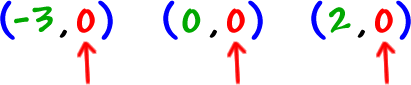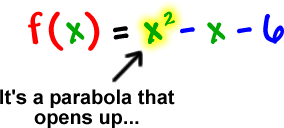Recall that a real zero is where a graph crosses or touches the x-axis.

Think of some points along the x-axis.What are the y values?  0!And remember that f(x) is just a fancy function name for y...

So, if we setand solve...

We get the real zeros!

Check it out:Let's see where it crosses the x-axis:So, the real zeros are

-2 and 3